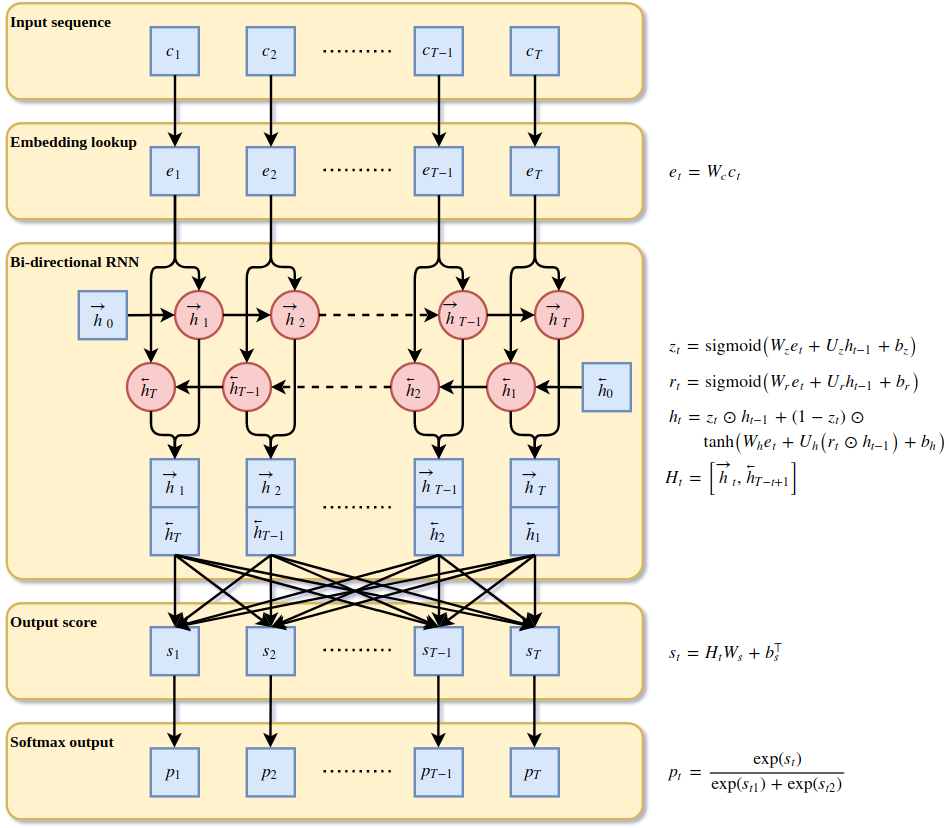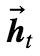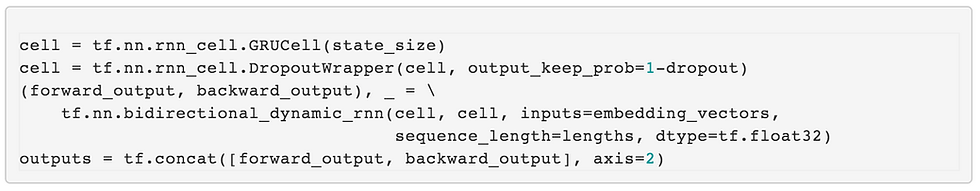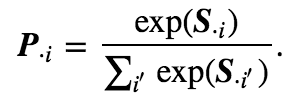top of page
• Sertis

# Thai word segmentation with bi-directional RNNIntroduction

In recent years, deep learning has provided state-of-the-art results in machine learning, with natural language processing (NLP) being no exception. The majority of development in NLP has been based on English and other well studied languages, mainly due to the availability of large, standardised corpuses. In contrast to this, we show an example of how to process Thai language.

Like many other East Asian languages such as Chinese and Japanese, words in Thai are typically written together without word boundary markers. For these languages, word segmentation is one of the first tasks in building applications of NLP such as topic classification, sentiment analysis, document similarity and chatbots. To illustrate the problem, the text below shows sentences in English with and without punctuation such as spaces and periods, which help to identify separate words.We human beings are able to read and understand the sentence without boundary markers easily, but for computers, processing such text is not straight forward. In Thai, there is no clear definition on what constitutes a word as it depends on the context, and writing down all possible rules is difficult. This sets a challenge which we approach with artificial neural networks (ANN) and deep learning.

Recurrent neural networks (RNN) are a special kind of ANN which has been one of the most successful models in NLP to date. We describe a simple word segmentation approach based on a RNN and provide Python code for TensorFlow 1.4 or newer, which is a popular framework from Google to build and train computational graphs. Broadly speaking, the model works by learning parameters in an ANN to provide rules to predict word boundaries. The weights are obtained by training the network iteratively by matching a sequence of characters in a sentence with a sequence of manually labelled word boundaries. As the training data, we use the InterBEST 2009 corpus with 148,995 sentences. With this corpus, we achieved an accuracy of 99.18% (𝐹₁ score), which is comparable to state-of-the-art.

Preprocessing

The corpus contains news, articles, encyclopedias and novels with words and sentences manually separated by marking the boundaries with special symbols. In the first step, found in file preprocess.py, we preprocess the corpus by separating each sentence into a list, creating an input sequence of characters by removing word boundary markers. We also create an output binary sequence, where 1 indicates beginning of a word (i.e. there should be a space before the word) and 0 indicates a character inside or at the end of a word (see figure below).

Each character in the input is mapped to a unique integer with a dictionary, which serves as a lookup table for converting between characters and input labels. The dictionary contains Thai alphabets (or abugidas to be specific), Latin alphabets, numerals and punctuation. The rest of the characters are mapped to an “unknown” label since these are deemed to contain no information required in the word segmentation.

The sentences are split into training and validation sets randomly by ratio 9:1 and are saved along with the sentence lengths to TFRecords format files. The format allows TensorFlow to access the data during training and validation of the model using a queue system.

Batching

The model is trained with batches of data for efficiency where each batch contains multiple sentences. Since the sentences are of different lengths, we must pad the input and output data to the maximum length 𝑇 determined by the longest sentence in the batch. The figure below illustrates how a batch of three input sentences corresponds to input and output label data provided to the model.Figure: A batch of three sentences which are converted to input and output labels and padded to the maximum sentence length in the batch. The top table contains the input characters, the left one corresponds to the dictionary values and the right one is the output labels of the word boundaries.

Model architecture

The architecture of the ANN model is shown in the figure below with a formulation of a single character at position 𝑡 in the single input sequence for each layer. Blue boxes indicate vectors and red circles GRU units in the RNN. In the following sections, we describe each layer in more detail. Source code for the model is found in file thainlplib/model.py which defines class ThaiWordSegmentationModel that includes methods for reading the data, building the model, validating the results and training the model. These are run from train.py.Figure: Artificial neural network architecture for Thai word segmentation. Vectors are indicated by blue boxes and GRU units by red circles. Each layer is described with corresponding formulation for a single character at position 𝑡 in the input sequence.

CHARACTER EMBEDDINGS

The first layer in the ANN learns character embeddings by mapping the discrete representation of each character label to a vector in continuous space. Perhaps, the best known approach to embeddings is word2vec for words, but a similar idea can be applied to characters as well. Each integer is turned into a one-hot encoded vector 𝑐 with length | 𝑐 | of the dictionary and multiplied by the embedding weights matrix 𝑊𝒸 of dimension | 𝑒 | x | 𝑐 |, i.e. 𝑒 = 𝑊𝒸𝑐. Since this operation effectively selects a row from the matrix, we may simply select the row corresponding to the integer directly to speed up computation. We can do this by using TensorFlow’s tf.nn.embedding_lookup function. The character embedding is implemented in the _build_embedding_rnn method of the ThaiWordSegmentationModel class:where the weights matrix 𝑊𝒸 is initialized uniformly from [-1,1]. The resulting embedding vector 𝑒 has length | 𝑒 | which corresponds to state size of the RNN cells in the next layer.

RECURRENT LAYER

In the second layer, we implement a bi-directional RNN to allow the capturing of dependencies between characters in both the forward and backward directions. In general, a RNN adds a recurrence to an ANN by taking 𝑋ₜ at position 𝑡 in a sequence and state vector ℎₜ–₁ from the previous step, where ℎ₀ is typically initialized with zeros. In this way, a RNN can use information from previous steps which is crucial to tasks where consecutive elements in a sequence share relevant information. In our case, we feed the embedding vectors [𝑒ₜ] ᵀₜ=1 of a sentence to the forward direction RNN and get 𝑇 state vectors [ℎₜ] ᵀₜ=1 of length | 𝑒 | from each step as output. The second component in the layer is the backward RNN which accounts for the dependencies that come after the character at index 𝑡 . This can be achieved simply by reversing the input sentence. TensorFlow provides a function tf.nn.bidirectional_dynamic_rnn to unroll the RNN dynamically at each training iteration to length 𝑇 .To clarify the notation, we denote the forward state vector byand the backward state vector byThe output matrix of the layer consists of the stacked state vectorsANNs are often trained with stochastic gradient descent or a related method. Weights in the ANN are adjusted based on their contribution to the prediction error using the backpropagation algorithm which iteratively finds the weights that minimize the error. However, vanilla RNNs suffer frequently from the vanishing gradient problem, where the amount of adjustment needed for the weights becomes very small due to many consecutive multiplications. This effectively prevents the training of weights further away from the error function as the tiny adjustments leave them virtually unchanged. A common solution to this problem is to replace the simple logic of vanilla RNNs with long short-term memory (LSTM) units. In our case, we computed the state vectors with gated recurrent units (GRU), which are faster to train due to the fewer number of parameters, but they have been said to be on a par with LSTM in performance. Formulas for a GRU are given in the model architecture figure.

The layer also contains dropout, which randomly selects different subset of weights in the GRU cells to not be trained. This is to avoid overfitting the model to the data, which is a common problem in deep learning. This is all put together and provided in the _build_embedding_rnn method:OUTPUT LAYER

The last layer is a fully connected network which provides a 𝑇x2 core matrix, 𝑆 = 𝐻𝑊ₛ + 𝑏ᵀₛ Each 𝑆ₜ is a score vector for the boundary and non-boundary labels, i.e. where the spaces should be put to separate the words. The weight matrix 𝑊ₛ has dimension 2| 𝑒 | x 2 and the bias vector 𝑏ₛ length 2. To obtain probabilities for the boundaries in the sentence for each label

𝑖 ⋲ ["boundary","non-boundary"] the scores are fed to the softmax functionSince there is a single degree of freedom, the last layer can provide just a single probability for each character. The implementation is provided in the _build_classifier method:Prediction error is measured with the cross entropy loss functionwhere 𝑦ᵢ contains the true boundaries of label 𝑖 in the sentence. The loss is calculated with tf.nn.sparse_softmax_cross_entropy_with_logits in TensorFlow. We do not want to include predictions for the padded characters in the total loss, so we remove them from 𝐿 by creating a mask with tf.sequence_maskand applying it with tf.boolean_mask. The loss per character for the sentence 𝑗 of length 𝑇ⱼis thenThe loss and the predicted sequences are returned by the _build_classifier method:Model training

The network is fed in a loop with batches of sentences randomly selected from the training dataset and trained with the Adam optimizer, which is an adaptive stochastic gradient descent (SGD) algorithm. The optimizer is defined in the _build_optimizer method:where global_step keeps track of the current training iteration. Adjustable hyperparameters include the state size of the GRU units, the learning rate of the optimizer and the dropout probability. These are all set in train.py. Batch size (number of sentences) usually equals the maximum number of sentences that the GPU memory can hold considering the length of the longest sentence.

To maximize utilization of the GPU, the data is read and prepared by the CPU in parallel while training the model with GPU using TensorFlow’s Dataset API. Reading the data is done with the _parse_record, _read_training_dataset, _read_training_dataset methods and initialization of the batch iterators over the data with _init_iterators.

Model validation

Performance of the word segmentation model is measured with the 𝐹₁ score, which is the harmonic mean of it’s precision and recall,Precision is the number of correctly predicted boundaries out of all boundaries (true and mispredicted) and recall is the number of correctly predicted boundaries out of all true boundaries. Since the 𝐹₁ measure is not differentiable while the optimization method requires differentiability, the cross entropy loss is used as a proxy. The 𝐹₁ score is calculated both for training and validation datasets to monitor potential overfitting.

Results and inference

We set the GRU state size to 128, learning rate to 0.001, dropout to 0.50 and batch size to 128 sentences and trained for 33,000 iterations overnight with a NVIDIA GeForce GTX 1070 – resulting in a precision of 99.04%, recall of 99.31% and 𝐹₁ score of 99.18% on the validation set. Training and validation losses are shown in the figure below and there appears to be no indication of overfitting. However, a more thorough test would be required to confirm this. There is an example in predict_example.py of how to infer word boundaries from input text.Figure: Training and validation losses at each iteration.

Bottom line

We applied word embeddings and a bi-directional RNN to Thai word segmentation. The relatively simple structure of the model provided results that are comparable to state-of-the-art approaches such as in Boonkwan et al. (2018) and Kittinaradorn et al. (2017). The strength of the bi-directional RNN is that it needs less feature engineering as opposed to convolutional neural networks (CNN) – another successful approach in deep learning which was used in Kittinaradorn et al. (2017). In the context of NLP, n-grams are usually constructed for CNNs to capture dependencies between nearby characters. The downside of a RNN is that it is slower to train than a CNN as it does not parallelize well due to it’s recurrent nature.

From here, there are multitudes of ways to improve the model, for example by preparing it for the types of texts that are not covered in the training data, and for spelling mistakes. Furthermore, the model could be extended to additionally learn higher level NLP tasks such as named entity recognition or speech tagging.

References:

• Boonkwan P., Supnithi T. (2018) Bidirectional Deep Learning of Context Representation for Joint Word Segmentation and POS Tagging. In: Le NT., van Do T., Nguyen N., Thi H. (eds) Advanced Computational Methods for Knowledge Engineering. ICCSAMA 2017. Advances in Intelligent Systems and Computing, vol 629. Springer, Cham

bottom of page